Courses
Courses for Kids
Free study material
Offline Centres
More

# Classification of Elements and Periodicity in Properties Class 11 Notes CBSE Chemistry Chapter 3 (Free PDF Download)Last updated date: 02nd Dec 2023
Total views: 677.1k
Views today: 12.76k

## Revision Notes for CBSE Class 11 Chemistry Chapter 3 (Classification of Elements and Periodicity in Properties) - Free PDF Download

Chemistry Class 11 Chapter 3 is crucial for Board and competitive examinations as it deals with chemical bonding and periodicity. Class 11 Chemistry Chapter 3 Notes by Vedantu explain the concepts of elements and periodic classification. Generally, an electronic configuration helps in classifying elements into a periodic table. Classification of Elements and Periodicity in Properties Class 11 Notes Details about the classification like d-block, p-block, s-block and f-block. This is based on metals and components which are arranged based on numbers. Ideally, the top elements are non-metals having a value less than 20.

## Topics Covered in Chapter 3 Classification of Elements and Periodicity in Properties of CBSE Class 11 Chemistry

Students looking for notes on Classification of Elements and Periodicity in Properties must know the topics covered in this chapter according to the latest syllabus prescribed by the CBSE. Below given are the topics covered in Chapter 3 Classification of Elements and Periodicity in Properties of CBSE Class 11 Chemistry.

1. Why do we need to classify elements?

2. Genesis of periodic classification

3. Modern Periodic Law and Present Form of the Periodic Table

4. Nomenclature of Elements with Atomic Numbers > 100

5. Electronic configuration of Elements and Periodic Table

• Electronic Configurations in Periods

• Groupwise Electronic Configurations

6. Electronic Configuration and Types of Elements: s-, p-, d- and f- Blocks

• The s-Block Elements

• The p-Block Elements

• The f-Block Elements (Inner-Transition Elements)

• The d-Block Elements (Transition Elements)

• Metals, Nonmetals and Metalloids

7. Periodic Trends in Properties of Elements

• Trends in Physical Properties (Atomic Radius, Ionic Radius, Ionisation Enthalpy, Electron Gain Enthalpy, Electronegativity)

• Periodic Trends in Chemical Properties

• Periodicity of Valence or Oxidation States

• Anomalous Properties of Second Period Elements

• Periodic Trends and Chemical Reactivity

Also, check CBSE Class 11 Chemistry revision notes for other chapters:

## Classification of Elements and Periodicity in Properties Chapter Related Important Study MaterialsIt is a curated compilation of relevant online resources that complement and expand upon the content covered in a specific chapter. Explore these links to access additional readings, explanatory videos, practice exercises, and other valuable materials that enhance your understanding of the chapter's subject matter.

 Classification of Elements and Periodicity in Properties Related Other Study Materials Classification of Elements and Periodicity in Properties Important Questions Classification of Elements and Periodicity in Properties NCERT Solutions Classification of Elements and Periodicity in Properties NCERT Exemplar

## Classification of Elements and Periodicity in Properties Class 11 Notes Chemistry - Basic Subjective Questions

### Introduction

A periodic table is a table that organises all known elements into groups based on their properties, with like elements grouped together in the same vertical column and dissimilar elements separated.

### Historical Development of Periodic Table

The atomic weights of the elements were used in all previous attempts to classify them.

Dobereiner defined triads as groups of three elements in 1829. A triad's chemical characteristics were comparable. The atomic weight of the middle element was found to be approximately equal to the arithmetic mean of the other two elements when the elements in a trio were ordered in order of increasing atomic weights.

 Triad Iron Cobalt Nickel Mean of 1st and 3rd Atomic weight 55.85 58.93 58.71 Atomic weights are nearly the same. Triad Lithium Sodium Potassium $\dfrac{{7 + 39}}{2} = 23$ Atomic Weight 7 23 39 Triad Chlorine Bromine Iodine $\dfrac{{35.5 + 127}}{2} = 81.25$ Atomic weight 35.5 80 127 Triad Calcium Strontium Barium $\dfrac{{40 + 177}}{2} = 88.5$ Atomic weight 40 87.5 137

### Newland’s Law of Octaves

When the lighter elements were placed in order of increasing atomic weights, the properties of every eighth element were comparable to those of the first, as the eighth note of a musical scale, according to an English chemist named John Alexander Newlands in 1865. Newlands' law of octaves was named after this generalisation.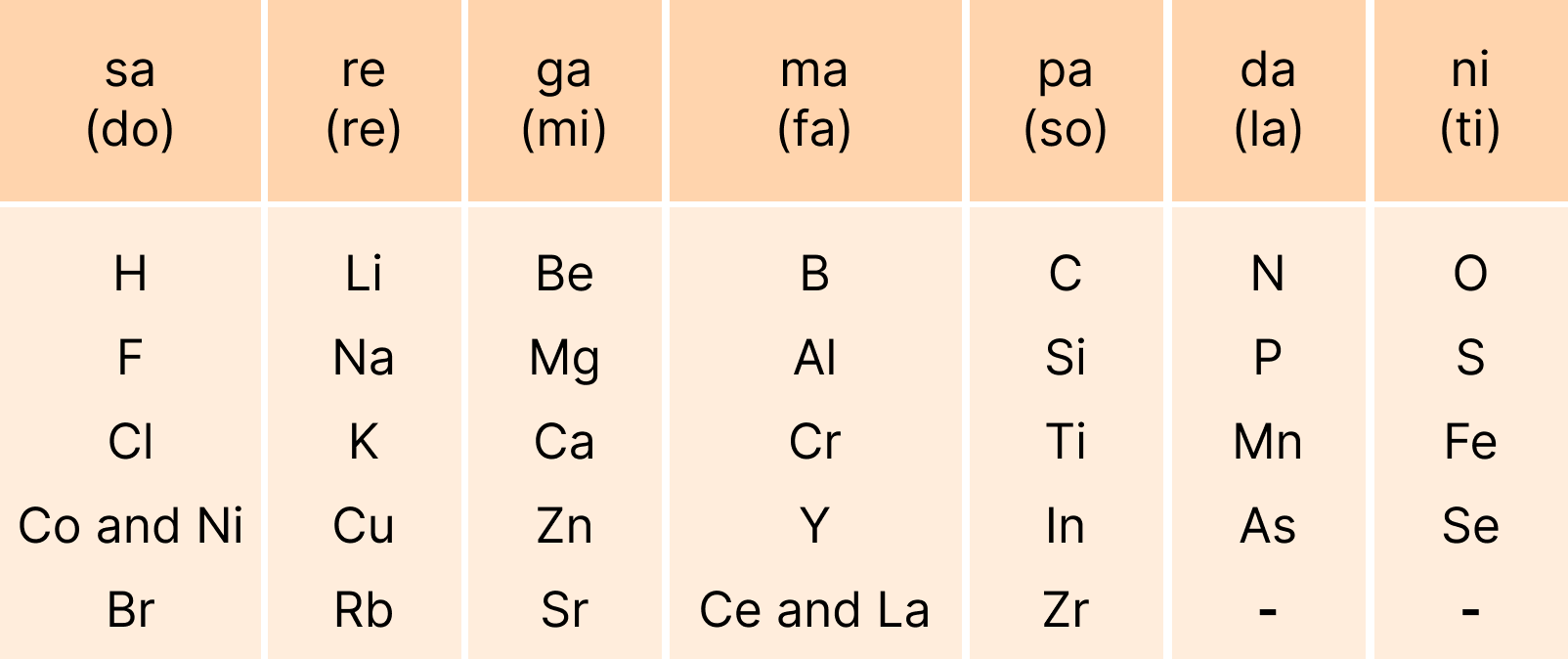### Lothar Meyer’s Curve

“The atomic masses of elements are periodic functions of their physical properties.”

According to Lothar Meyer, atoms with similar properties occupy similar places in the atomic volume, as measured by the atomic mass curve.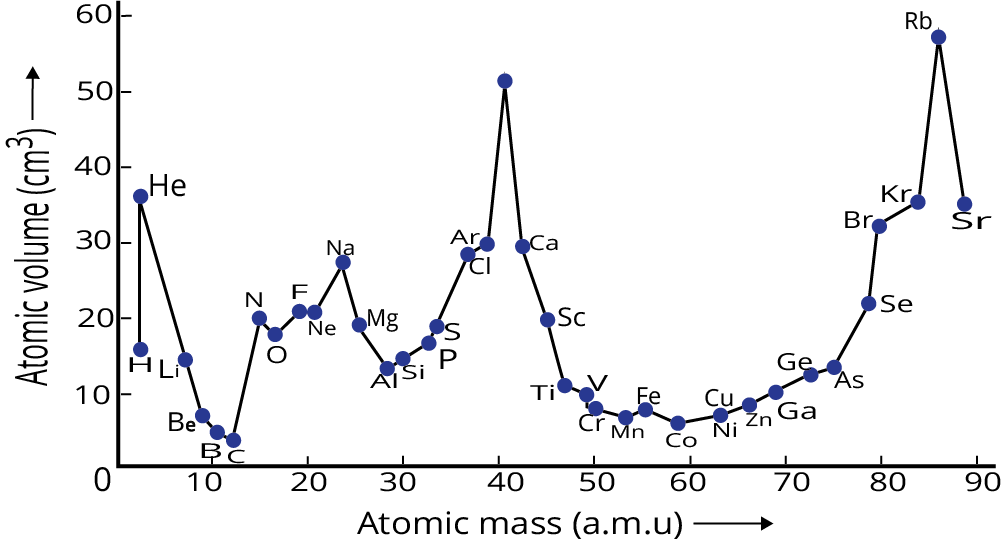### Mendeleev’s Periodic Law

Mendeleev organised atoms in a table's horizontal rows and vertical columns in order of increasing atomic weights, so that elements with comparable properties were grouped together in the same vertical column.

### Modern Periodic Law

Henry Moseley, an English physicist, discovered regularities in the distinctive X-ray spectra of the elements in 1913. Instead of a straight line between $\sqrt v$ versus atomic mass, a plot of $\sqrt v$  (where $v$  is the frequency of X-rays emitted) against the atomic number (Z) produced a straight line.

As a result, Mendeleev's Periodic Law was altered correspondingly. The Modern Periodic Law can be stated as follows: The physical and chemical properties of elements are periodic functions of their atomic numbers.

### Periodic Table of Elements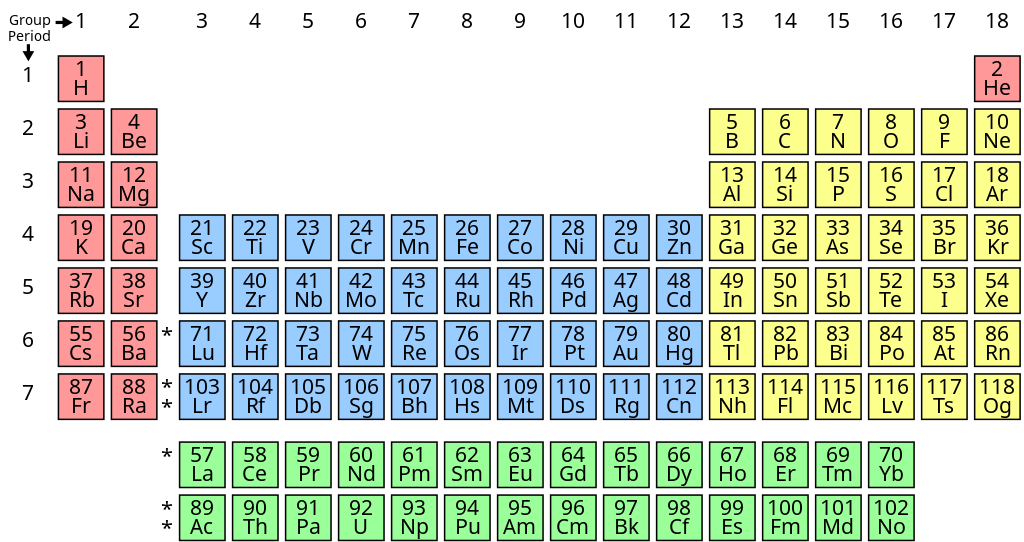Nomenclature of Elements with Atomic numbers > 100

The authority of naming new elements had hitherto been reserved for the discoverer, and the recommended name was validated by the IUPAC.

Table: Notation for IUPAC Nomenclature of Elements

 Digit Name Abbreviation 0 Nil n 1 Un u 2 Bi b 3 Tri t 4 Quad q 5 Pent p 6 Hex h 7 Sept s 8 Oct o 9 enn e

Table: Nomenclature of Elements with Atomic Number above 100

 Atomic Number Name Symbol 101 Unnilunium Unu 102 Unnibium Unb 103 Unniltrium Unt 104 Unnilquadium Unq 105 Unnilpentium Unp 106 Unnilhexium Unh 107 Unnilseptium Uns 108 Unniloctium Uno 109 Unnilennium Une 110 Ununnillium Uun

### Prediction of Block, Period, and Group

1. What electronic configuration

2. Block - last electron enters into which orbital

3. Period - Maximum value of principal quantum number

Group - $s$ - block - number of valence electron

$p$ - block - 10 + number of valence electron

$d$ - block – ns + number of $(n - 1)d$ electrons

$f$ - block – ${\text{III B}}$

### Properties of an Element

We can't measure an isolated atom's exact size since its outermost electron has a slim possibility of being located far away from the nucleus. So, depending on the atoms' surroundings, multiple forms of atomic radius can be utilised, such as covalent radius, van der Waals' radius, and metallic radius.

The covalent radius of a molecule is half the distance between the nuclei of two identical atoms linked by a single covalent bond.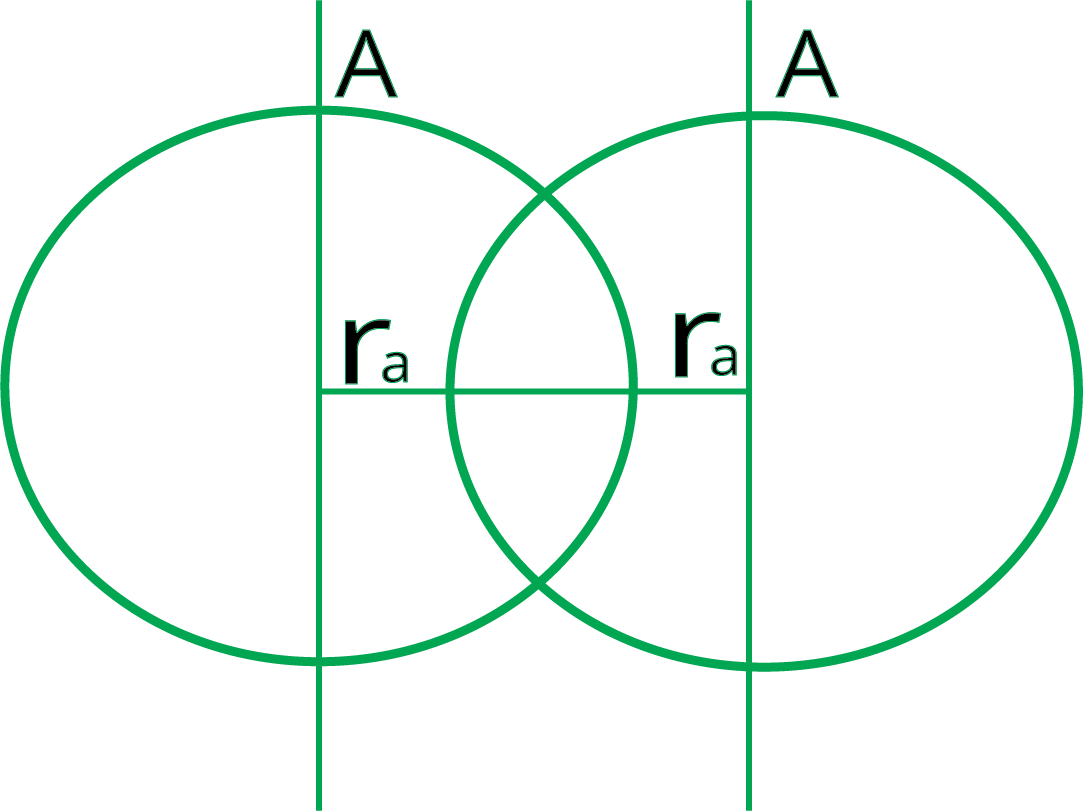So, covalent radius for ${\text{A}} - {\text{A}}$

${r_A} = \dfrac{{{d_{A - A}}}}{2}$

If covalent bond is formed between two different elements then

${d_{A - B}} = {r_A} + {r_B} - 0.09({\chi _A} - {\chi _B})$

Where, ${\chi _A}$ and ${\chi _B}$  are electronegativities of A and B.

In the solid state, it is half of the internuclear distance between adjacent atoms of two neighbouring molecules.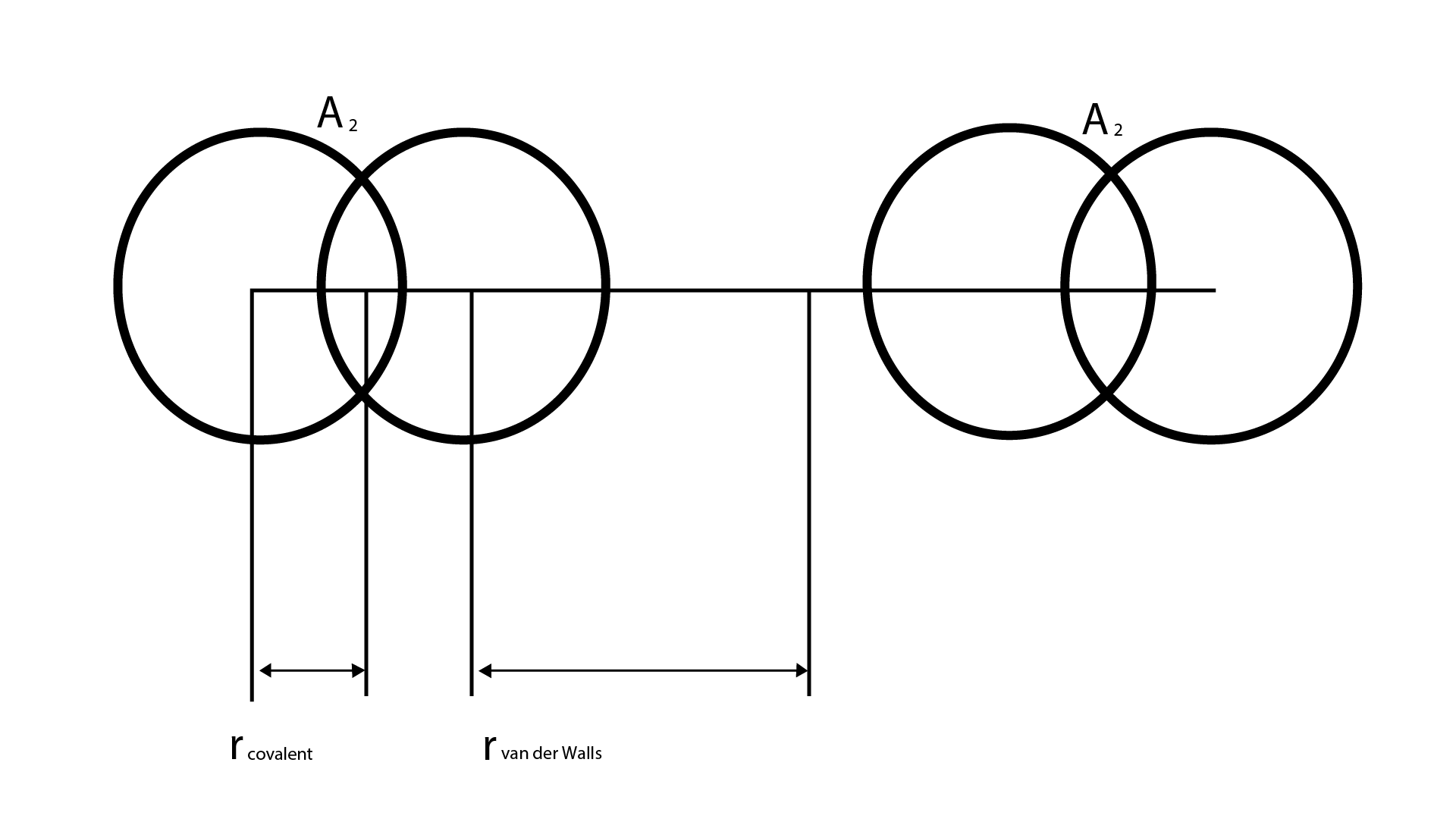${r_{vander\;waal}} = \dfrac{{{d_{A - A}}}}{2}$

In the metallic crystal lattice, it is half the distance between the nuclei of two neighbouring metal atoms.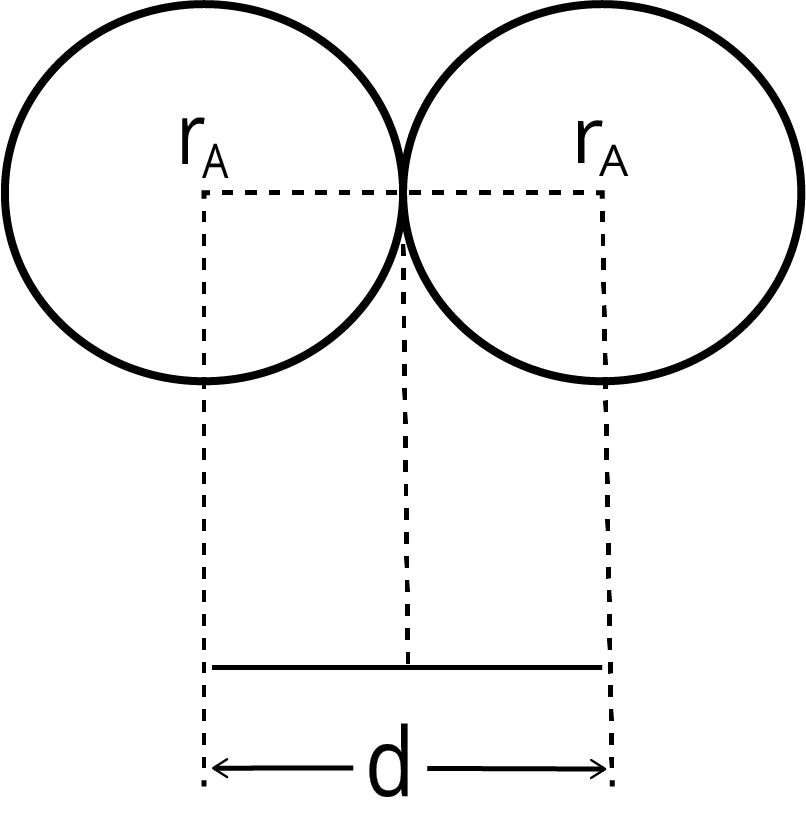So, metallic radius for ${\text{A}} - {\text{A}}$ :

$d = {r_A} + {r_A}$

${r_A} = \dfrac{d}{2}$

Therefore: ${r_{{\text{covalent}}}} < {r_{{\text{metallic}}}} < {r_{{\text{vander}}\;{\text{waal}}}}$

### Variation of Atomic Radii in the Periodic Table

As we move from left to right in a period, the covalent and van der Waals radii generally decrease with increasing atomic number.

1. Variation Along a Period: Because the outer electrons are in the same valence shell throughout the period, the effective nuclear charge increases as the atomic number grows, increasing the attraction of electrons to the nucleus.

2. Variation Along with a Group: The atomic radius of a group grows as the number of atoms in the group grows. The reason for this is that as the primary quantum number $(n)$  increases inside the group, the valence electrons become further away from the nucleus.

3. Ionic Radius: A cation is formed when an electron is removed from an atom, and an anion is formed when an electron is gained. In general, element ionic radii follow the same pattern as atomic radii. Because it has fewer electrons than its parent atom, a cation is smaller, but its nuclear charge remains the same. Because the addition of one or more electrons causes enhanced electron repulsion and a decrease in effective nuclear charge, the size of an anion will be bigger than that of the parent atom. The ionic radius of the fluoride ion $({{\text{F}}^ - })$ , for example, is 136 pm, while the atomic radius of fluorine is just 64 pm. The atomic radius of sodium, on the other hand, is 186 pm, whereas the ionic radius of $N{a^ + }$  is 95 pm.

4. Isoelectronic Species: Isoelectronic species have the same number of electrons as each other. ${O^{2 - }}$ , ${F^ - }$ , $N{a^ + }$ , and $M{g^{2 + }}$ , for example, all have the same number of electrons (10). Because of their various nuclear charges, their radii would be varied. Because the electrons are more attracted to the nucleus, the cation with the higher positive charge will have a smaller radius. The radius of an anion with a higher negative charge will be larger. The net repulsion of the electrons will outweigh the nuclear charge in this situation, causing the ion to expand in size.

Order of Atomic radii is: $M{g^{2 + }} < N{a^ + } < {F^ - } < {O^{2 - }}$

### General Trend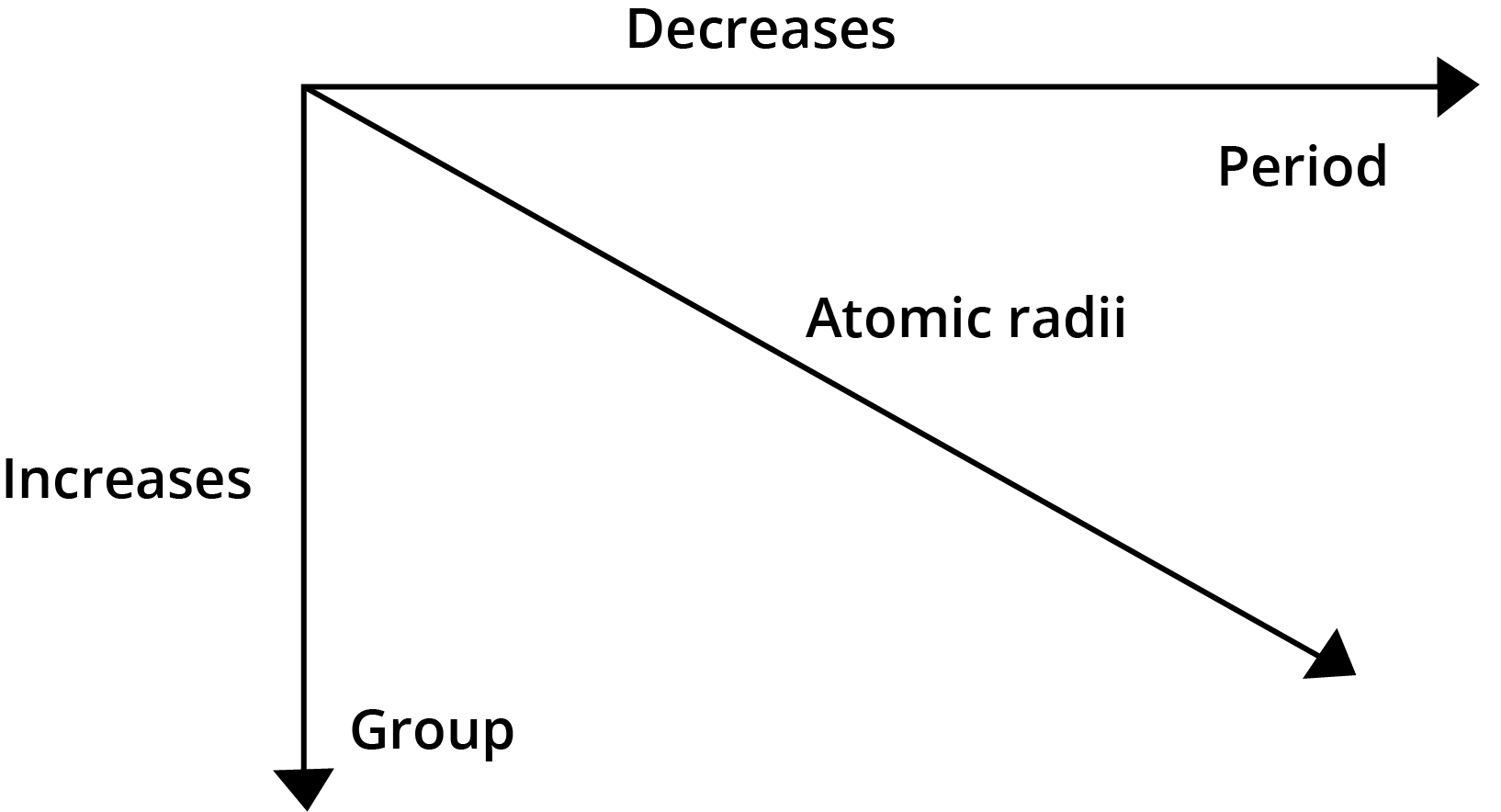### Ionization Energy

Ionization energy is the least amount of energy required to remove an electron from the outermost orbit of an isolated atom in the gaseous state.

$M\xrightarrow[{ - {e^ - }}]{{I{E_1}}}{M^ + }\xrightarrow[{ - {e^ - }}]{{I{E_2}}}{M^{2 + }}\xrightarrow[{ - {e^ - }}]{{I{E_3}}}{M^{3 + }}\xrightarrow[{ - {e^ - }}]{{I{E_4}}}{M^{4 + }}$

$I{E_1}$ , $I{E_2}$ , $I{E_3}$ and $I{E_4}$ are successive ionization energies.

$I{E_4} > I{E_3} > I{E_2} > I{E_1}$ or ${\Delta _i}{H_4} > {\Delta _i}{H_3} > {\Delta _i}{H_2} > {\Delta _i}{H_1}$

Variation of Ionisation Energy in Periodic Table

1. Variation Along a Period: The value of ionisation enthalpy grows from left to right over time, with intervals where the atoms' configurations are somewhat stable. Increased nuclear charge and decreased atomic radii are simple explanations for the observed trends. Both factors enhance the force of attraction towards the nucleus, requiring more and more energy to remove the electrons, resulting in higher ionisation enthalpies.

2. Variation Along a Period: The atomic size grows steadily as the group progresses due to the addition of one additional primary energy shell at each consecutive element. As a result, the ionisation enthalpy value drops as the force of attraction towards the valence electrons decreases.

### Units of I.E/I.P

It is expressed in electron volts $(eV)$  per atom, kilocalories per mole $(kcal\;mo{l^{ - 1}})$ , or kilojoules per mole $(kJ\;mo{l^{ - 1}})$  units. The energy gained by an electron while travelling under a potential difference of one volt is known as an electron volt.

1 electron volt per atom:

$= 3.83 \times {10^{ - 20}}\;cal\;per\;atom$

$= 1.602 \times {10^{ - 19}}\;J\;per\;atom$

$= 3.83 \times {10^{ - 20}} \times 6.023 \times {10^{23}}\;cal\;mo{l^{ - 1}}$

$= 23.06\;kcal\;mo{l^{ - 1}}$

$= 1.602 \times {10^{ - 19}} \times 6.023 \times {10^{23}}\;J\;mo{l^{ - 1}}$

$= 96.49\;kJ\;mo{l^{ - 1}}$

1 electron volt per atom = $23.06\;kcal\;mo{l^{ - 1}}$ = $96.49\;kJ\;mo{l^{ - 1}}$

### Important Points

• The ionisation energy of an atom or an ion increases as the size of the atom or ion decreases.

• As the screening effect decreases, the ionisation energy increases.

• With the increased nuclear charge, ionisation energy rises.

• If an atom's orbitals are half filled or entirely filled, the ionisation energy rises.

• The penetrating power of orbitals is in the order: $s > p > d > f$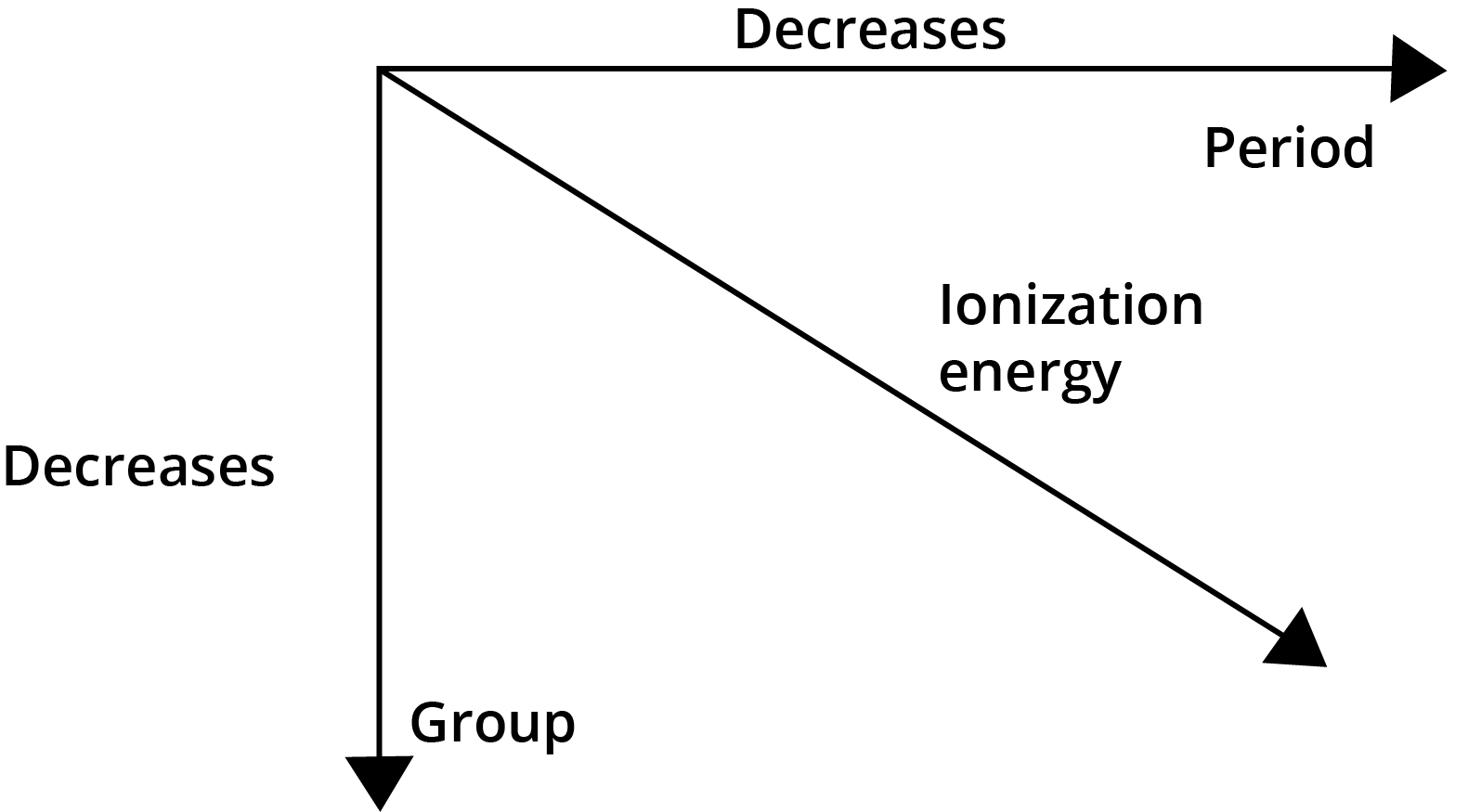### Electron Gain Enthalpy

The Electron Gain Enthalpy is the enthalpy change that occurs when an electron is added to a neutral gaseous atom $(X)$  to turn it into a negative ion. The ease with which an atom adds an electron to create an anion is measured by the electron gain enthalpy.

$X(g) + {e^ - } \to {X^ - }(g)$

The process of adding an electron to an atom can be either endothermic or exothermic, depending on the element. When an electron is added to an atom, energy is dissipated, and the electron gain enthalpy is negative for many elements. Because they can achieve stable noble gas electrical configurations by taking up an electron, group 17 elements (the halogens) have exceptionally high negative electron gain enthalpies. Noble gases, on the other hand, have substantial positive electron gain enthalpies because the electron must enter the next higher main quantum level, resulting in an electronic configuration that is exceedingly unstable.

### Variation of Electron Gain Enthalpy

1. Variation Along a Period: In a period, the electron gain enthalpy shifts from left to right, becoming increasingly negative. As the atomic number increases over time, the size of the nucleus shrinks and the nuclear charge increases. Due to the larger force of attraction by the nucleus for the incoming electron, both of these considerations favour the addition of an extra electron.

2. Variation Along with a Group: Going down from top to bottom in a group, the electron gain enthalpies become less negative. This is owing to the fact that as you move down a group, your size grows. In comparison to the other factor, which is an increase in nuclear charge, this factor is more important.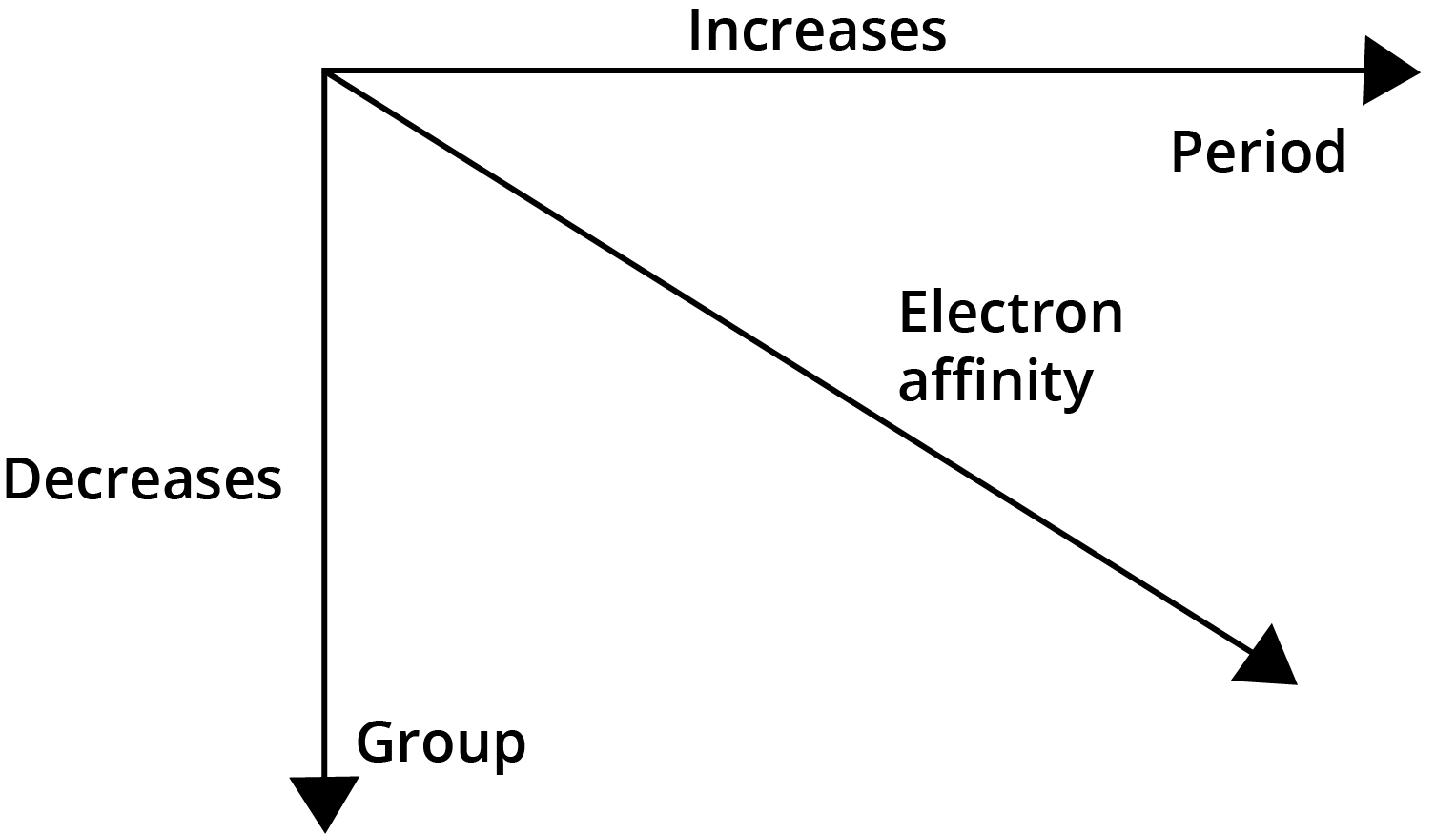### Electronegativity

Electronegativity refers to an atom's inclination to draw the shared pair of electrons towards itself. The electronegativity of fluorine is 4.0, according to Pauling, and the electronegativity of other elements can be derived as:

$({\chi _A} - {\chi _B}) = 0.208{[{E_{A - B}} - {({E_{A - A}} \times {E_{B - B}})^{1/2}}]^{1/2}}$

According to Mulliken:

Electronegativity = $\dfrac{{{\text{IP + EA}}}}{2}$

Where, IP is the Ionization potential and EA is the Electron Affinity

If IP and EA are taken as electron volt:

• Percentage ionic character = $16({\chi _A} - {\chi _B}) + 3.5{({\chi _A} - {\chi _B})^2}$ , where ${\chi _A}$  and ${\chi _B}$  are electronegativities of A and B.

• If the difference in the electronegative of combining atoms is 1.7, the bond is 50% covalent and 50% ionic.

• The oxide takes on a basic character when the difference between the electronegativities of oxygen and the element is quite large.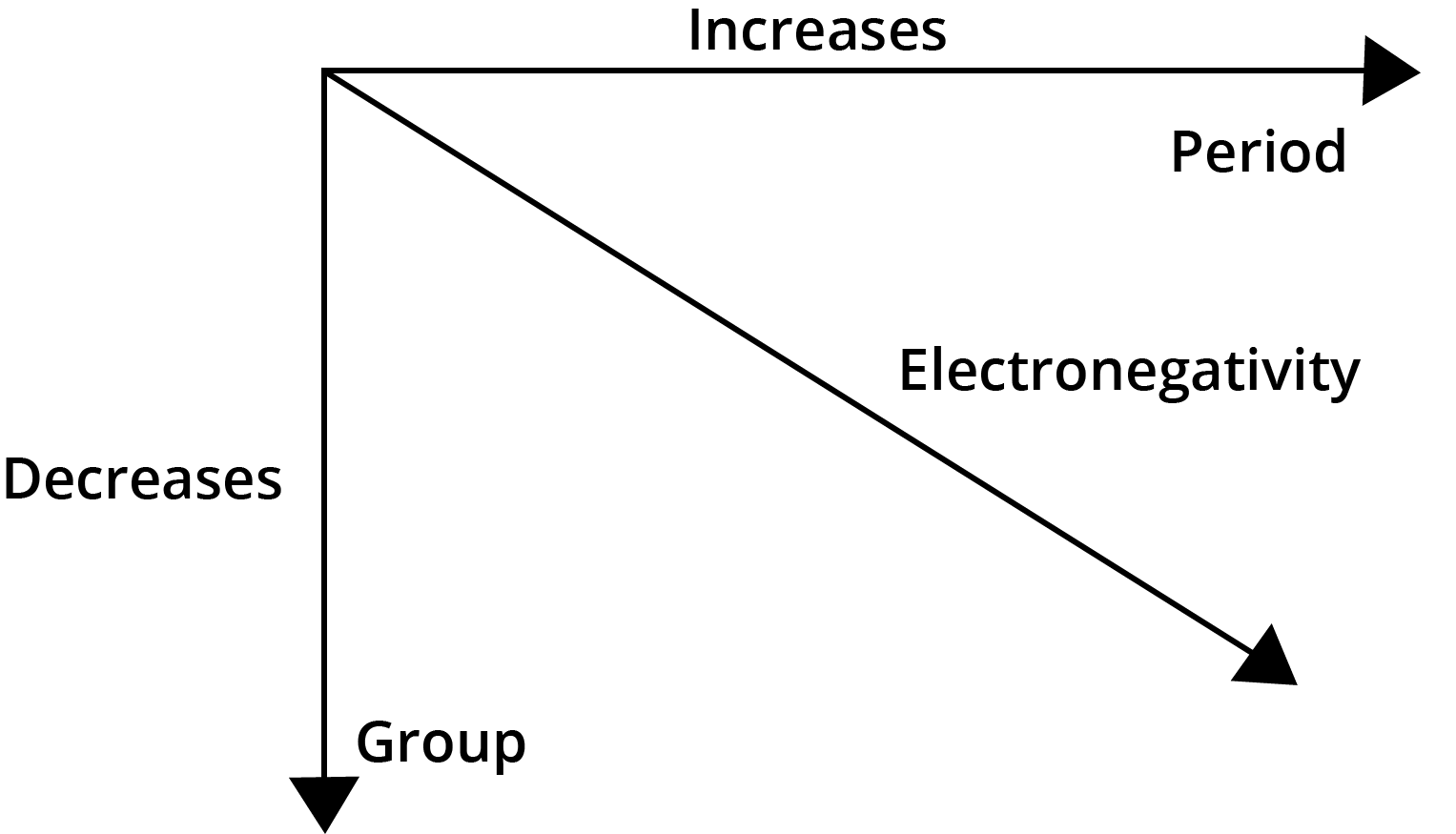### The Periodic Trends of Elements in the Periodic Table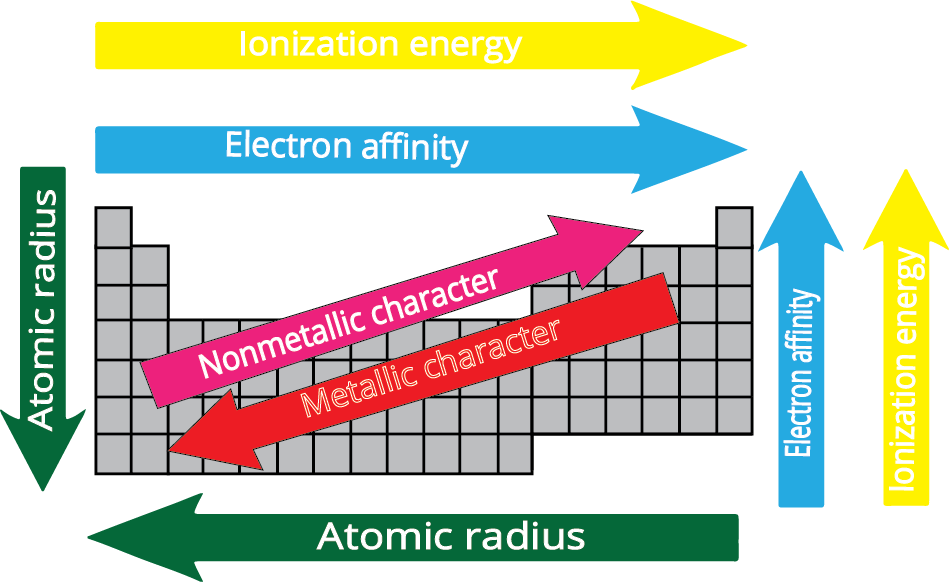### Periodicity of Valence or Oxidation States

Valence electrons are the electrons found in an atom's outermost shell, and the number of these electrons determines the atom's valence or valency. Because of this, the atom's outermost shell is also known as the valence shell, and the orbitals that make up the valence shell are known as valence orbitals. The valence of an atom is generally equal to either the number of valence electrons ($s$ - and $p$ - block elements) or eight minus the number of valence electrons in representative elements.

 Group 1 2 13 14 15 16 17 18 Number of Valence electrons 1 2 3 4 5 6 7 8 Valence Shell 1 2 3 4 3, 5 2, 6 1, 7 0, 8

Transition and inner transition elements, on the other hand, have varying valence due to the presence of not just valence electrons but also $d$ - or $f$ - electrons. Their most common valences, however, are 2 and 3.

Let us now look into valence periodicity over time and within a group.

1. Variation Along a Period: The number of valence electrons grows from 1 to 8 as we walk through a period from left to right. However, the valence of elements in relation to hydrogen or oxygen increases from 1 to 4 before decreasing to zero. The more electronegative oxygen receives two electrons from each of the two sodium atoms in the production of the $N{a_2}O$  molecule, giving it an oxidation state of –2. Sodium, on the other hand, loses one electron to oxygen and has an oxidation state of +1 because of its valence shell electronic configuration of $3{s^1}$ . Thus, an element's oxidation state in a compound can be described as the charge obtained by its atom as a result of the electronegativity of the other atoms in the molecule.

2. Variation Within a Group: Because the number of valence electrons remains constant as we proceed down the group, all elements in a group have the same valence. All elements in group 1 (alkali metals), for example, have valence one, whereas all elements in group 2 (alkaline earth metals) have valence two. Noble gases of group 18 have zero valances, which means their valence is zero because they are chemically inert.

### Anomalous Properties of Second Period Elements

Some elements from the second period appear to be identical to elements from the third period when put diagonally next to each other while belonging to separate groups. Lithium (group 1), for example, resembles magnesium (group 2), while beryllium (group 2) resembles aluminium (group 13), and so on. A diagonal relationship refers to the resemblance in attributes of elements that are arranged diagonally next to each other.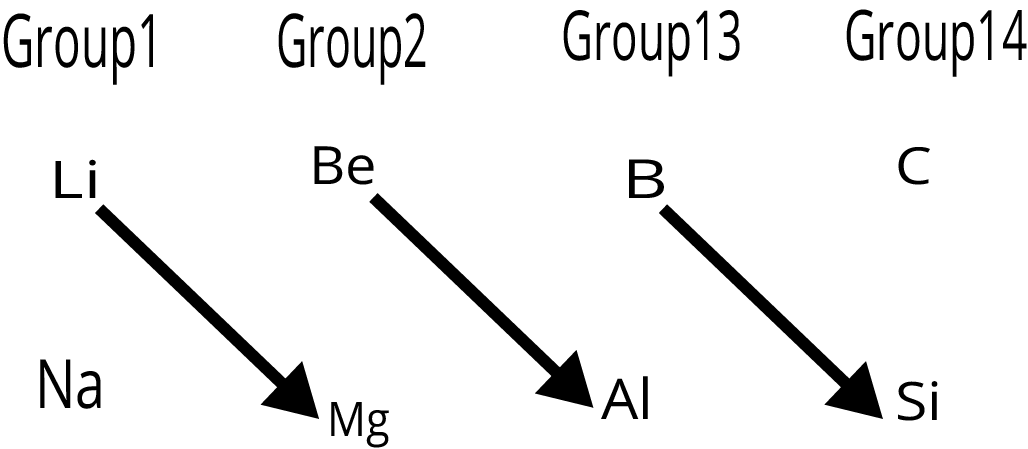The elements' unusual behaviour is caused by their short size, huge charge/radius ratio, and strong electronegativity. Furthermore, the first member of the group only has four valence orbitals ($2s$  and $2p$ ) accessible for bonding, whereas the second member of the group has nine $(3s,3p,3d)$. As a result, the maximum covalency of the first member of each group is 4 (for example, boron can only create ${[B{F_4}]^ - }$ ), whereas the other members of the groups can expand their valence shell to include more than four pairs of electrons (for example, aluminium can form ${[Al{F_6}]^{3 - }}$ ). Furthermore, as compared to later members of the same groups, the first member of p-block elements has a larger potential to make p-p multiple bonds to itself (e.g., $C = C$ , $C \equiv C$ , $N = N$ , $N \equiv N$ ) and to other second period elements (e.g., $C = O$ , $C = N$ , $C \equiv N$ , $N = O$ ).

### Reactivity of Metals

The ability of metals to lose electrons from their outermost shell is used to determine their reactivity.

In a period: Going from left to right in a period, an element's tendency to lose electrons decreases. As a result, metal reactivity reduces as you move from left to right. The reactivity of third-period elements, for example, follows the order: $Na > Mg > Al$

In a group: As we move down the group, the tendency to lose electrons rises. As a result, metal reactivity rises as one moves down the group. As a result, the reactivity in group 1 follows the order: $Li < Na < K < Rb < Cs$

### Reactivity of Non – Metals

The likelihood of a non-metal to gain electrons and form an anion is used to determine its reactivity.

In a period: Non-metals' reactivity rises from left to right over time. Non-metals tend to produce anions during reactions. The reactivity of non-metals, for example, increases in order in the second phase. $C < N < O < F$

In a group: As we move down the group, the reactivity of non-metals declines. This is due to the fact that as one moves down the group, the inclination to accept electrons decreases. The order of halogen reactivity is as follows: $F > Cl > Br > I$

The basic normal oxide generated by the element on the far left (e.g., $N{a_2}O$ ) is the most basic, whereas the acidic normal oxide formed by the element on the far right is the most acidic (e.g., $C{l_2}{O_7}$ ). The oxides of the elements in the centre are either amphoteric ($A{l_2}{O_3}$ , $A{s_2}{O_3}$ ) or neutral ($CO$ , $NO$ , ${N_2}O$ ). Amphoteric oxides are acidic with bases and basic with acids, whereas neutral oxides are neither acidic nor basic.

### Inert Pair Effect

As we progress through groups 13-16, the tendency of valence shells-electrons to participate in bond formation declines. Lower oxidation states become more stable as a result of this.

Reason: The effect of the increasing nuclear charge overcomes the effect of the equivalent rise in atomic size as we move down these groups. As a result, the $s$ -electrons become more securely held (penetrating) and hence less willing to participate in bond formation. The lower oxidation state becomes more stable as a result.

### Summary and Important Points to Remember

1. The periodic table of Mendeleev was based on the atomic masses of the elements. Only 63 elements were known when Mendeleev presented the periodic chart. In the table, he left 29 spots for unknown elements.

2. The atomic numbers of the elements are used in the modern Mendeleev periodic table. “The physical and chemical properties of the elements are a periodic function of their atomic numbers,” says the modern periodic law. A period is a horizontal row in the periodic table, and a group is a vertical column. The contemporary Mendeleev periodic table has seven periods and nine groups.

3. Seven eras and eighteen vertical columns make up the long or expanded periodic table (groups or families). A period's elements all have the same number of energy shells, or principal quantum number $(n)$. These are numbered one through seven. There are now 114 elements known. The elements in a vertical column (group) have comparable valence shell electrical configurations and, as a result, chemical characteristics.

4. The $s$ -, $p$ -, $d$ -, and $f$ - blocks of elements are named for the orbital that receives the last electron. These blocks' electronic configuration is as follows:

$s$ - block: [Noble gas] $n{s^{1 - 2}}$ , but hydrogen has $1{s^1}$ configuration.

$p$ - block: [Noble gas] $n{s^2}n{p^{1 - 6}}$

$d$ - block: [Noble gas] $(n - 1){d^{1 - 10}}n{s^{1 - 2}}$

$f$ - block: [Noble gas] $(n - 2){f^{1 - 14}}(n - 1){d^{0 - 1}}n{s^2}$

1. The ingredients are classified into three categories:

(i). Metals account for more than 78% of all known elements. Metals are the $s$ -block, $d$ - block, and $f$ - block elements. Metals make up the higher components of the $p$ - block.

(ii). The number of non-metals is less than twenty. (Non-metals include $C$ , $N$ , $P$ , $O$ , $S$ , $Se$ , $H$ , $F$ , $Cl$ , $Br$ , $I$ , $He$ , $Ne$ , $Ar$ , $Kr$ , $Xe$ , and $Rn$ .)

(iii). Semimetals or metalloids are elements that exist on the boundary between metals and nonmetals. Metalloids include $B$ , $Si$ , $Ge$ , $As$ , $Sb$ , $Te$ , $Po$ , and $At$ .

1. The IUPAC has devised a new mechanism for giving newly discovered elements a temporary name. The name is derived directly from the element's atomic number. The names of the elements from atomic numbers 104 to 110 have been accepted by IUPAC.

2. Periodicity is defined as the occurrence of identical properties of the elements at regular intervals when the elements are grouped in the periodic table in order of increasing atomic numbers. The repetition of similar electronic configurations of the atom in the valence shell after certain specified periods is the cause of periodicity. These definite intervals are 2, 8, 8, 18, 18 and 32. These are referred to as "magic numbers." Periodicity can be seen in a variety of qualities that are either directly or indirectly related to the electronic configuration.

(i). Across a period, the effective nuclear charge increases.

(ii). Atomic radii tend to decrease as time passes.

(iii). As you progress through the groupings, the atomic radii tend to rise.

(iv). There are three types of atomic radius:

1. Covalent radius: The distance between the nuclei of two comparable atoms linked by a single covalent bond is half of this radius. Non-metals are typically described in this way.

2. Crystal or metallic radius: In the metallic lattice, it is half of the internuclear distance between the two closest atoms. It's a term that's commonly applied to metals.

3. Van der Waals’ radius: In the solid-state, it is half of the internuclear distance between the nearest atoms of two neighbouring molecules.

For an atom:

• Anions are typically larger than cations.

• Cations are smaller and anions are larger than the elements' neutral atoms.

Cation size < Neutral atom size < Anion size

• Due to lanthanide contraction, elements in the 2nd and 3rd transition series belonging to the same vertical column are comparable in size and characteristics.

• The first element is that each group of representative elements has aberrant features, i.e., it varies from the other elements in the group due to the atom's considerably smaller size.

• Isoelectronic ions are ions with the same amount of electrons but a different nuclear charge. The size of isoelectronic ions reduces as the atomic size grows, i.e., the larger the nuclear charge, the smaller the ion.

• Ionisation enthalpy is the amount of energy necessary to remove the most loosely held electron from a gaseous isolated atom.

• The values of ionisation enthalpy tend to rise over time.

• The enthalpy of ionisation decreases as you progress through the group.

• Higher energy is required to remove electrons from filled and half-filled shells. The ionisation enthalpy of nitrogen, for example, is larger than that of oxygen. High values are found in $Be$, $Mg$, and noble gases.

• Ionisation enthalpy values in metals are low, but non-metals have large ionisation enthalpy values.

• The values of successive ionisation enthalpies of an atom are higher. $I{E_1} < I{E_2} < I{E_3}.....$

• Electron gain enthalpy is the enthalpy change that occurs when an electron is added to an isolated gaseous atom of an element. The first electron gain enthalpy of most elements is negative since energy is released during the process, but in the case of atoms with stable configurations such as $Be$ , $Mg$ , $N$ , noble gases, and so on, the values are positive or near zero.

• From left to right in a period, electron gain enthalpy grows more negative, and from top to bottom in a group, it becomes less negative.

• The enthalpies of successive electron gains are always positive.

• Negative electron gain enthalpy is higher in elements with higher ionisation enthalpy.

• An atom's tendency to attract the shared pair of electrons in a bond is known as electronegativity.

• Electronegativity rises over the periods and falls as you progress through the groups.

• Metals have low electronegativities, while non-metals have high ones.

• Metallic character decreases with time and increases as the group progresses.

• The elements' reducing nature decreases over time, whereas their oxidising nature increases.

• Moving from left to right in a period, the basic character of the oxides decreases while the acidic character grows.

### Some Important Facts About Elements

• At room temperature, bromine is a non-metal that is liquid.

• At ambient temperature, mercury is the only metal that is liquid.

• Metals with low melting points include gallium (m.pt. ${29.8^ \circ }C$ ), caesium (m.pt. ${28.5^ \circ }C$ ), and francium (m.pt. ${27^ \circ }C$ ).

• Tungsten ${\text{(W)}}$  is the metal with the highest melting point ${3380^ \circ }C$ .

• Among nonmetals, carbon has the greatest melting point ${4100^ \circ }C$ .

• The most prevalent element on the planet is oxygen.

• The most plentiful metal is aluminium.

• The transition metal iron is the most common.

• Osmium $(22.57\;g\;c{m^{ - 3}})$  and iridium $(22.61\;g\;c{m^{ - 3}})$  have the highest density.

• The lightest metal is lithium. It has a density of $0.54\;g\;c{m^{ - 3}}$ .

• The best conductor of electricity is silver.

• The hardest natural substance is diamond (carbon).

• The atomic volume of francium is the largest.

• Boron has the smallest atomic volume of all the elements.

• Nitrogen is the most abundant gas in the atmosphere.

• Fluorine is the most electronegative element in the periodic table.

• Chlorine has the highest negative electron gain enthalpy of any element.

• The ionisation enthalpy of helium is the highest.

• The ionisation enthalpy of cesium or francium is the lowest.

• The smallest and largest atoms are helium and francium, respectively.

• The smallest and largest anions are ${H^ - }$  and ${I^ - }$  ions, respectively.

• The smallest and largest cations are ${H^ + }$  and $C{s^ + }$ , respectively.

• The most electropositive element is Cesium.

• Phosphorus, ${P_4}$ , is an element that is preserved in water (white or yellow).

• $Na$ , $K$ , $Rb$ , $Cs$  and other elements are stored in kerosene.

• Iodine is the element that transcends.

• The most prevalent element in the universe is hydrogen.

• The only coloured gas with a garlic odour is ozone.

• Electronegativity values for metalloids are closer to 2.0.

• Technetium is the first synthetic (i.e., man-made) element (At. No. 43).

• Plutonium is the most toxic metal.

• Astatine is the rarest element in the earth's crust.

• Transuranic elements are the elements that come after uranium. Trans-actinides, or super heavy elements, are those with Z =104–112,114, and 116. All of these elements are synthetic, meaning they were created by humans. These are radioactive elements that do not occur naturally.

• Ruthenium $(Ru)$ , germanium $(Ge)$ , polonium $(Po)$ , and americium $(Am)$  are elements named for the countries Ruthenia (Russia), Germany, Poland, and America, respectively.

• Rutherfordium $(Rf,104)$  is also known as Kurchatovium $(Ku)$ , and dubnium $(Db,105)$  is also known as hahnium.

• Promethium $(Pm,61)$ , a lanthanide element, does not exist in nature. It's a man-made substance.

• Members of these groups are given special names in the periodic table.

 Group 1 Or I A Alkali metals Group 2 Or II A Alkaline earth metals Group 15 Or V A Pnicogens Group 16 Or VI A Chalcogens Group 17 Or VII A Halogens Group 18 Or VIII A Inert or noble gases

Chemistry notes on Class 11 Chapter 3 PDF specify the metallic characteristics and increase of atomic numbers. This decrease is observed from left to right, while the physical and chemical properties start altering in nuclear numbers. Students by a thorough reading of Chemistry Chapter 3 Class 11 Notes will understand that elements of the similar group start showing alike chemical properties. This is caused by the presence of valence shell in an electronic arrangement. You can also choose to download these notes for free of cost to study st your own convenience.

Apart from understanding the definition of elements and period, young learners need to learn specific topics. This notes of Chapter 3 Of Chemistry Class 11 have listed the vital terms crucial for examination. Take a look!

### Important Terms Determining Periodic Tendency in Element Properties

1. Atomic Sizes: The nuclear radii of a group directly increase when an atomic number increase. This atomic radius usually downgrades from left to right in a period.

2. Ionisation Enthalpies: This is a complex periodic tendency which along a group decreases, but increases along the period across it.

3. Electron Gain Enthalpies: It makes a period negative than lowering negativity in a group.

4. Electronegativity: This phenomenon that reduces in a group but starts to rise across a period.

5. Chemical Reactivity: It has the highest centre which is present at the two extremes in a period.

Chemistry Class 11 Chapter 3 Notes explain these phenomena, which form a chemical reaction based on element property. There are also structural characteristics present in the Ch 3 Chemistry Class 11 Notes which are vital to grasp.

### Terms Related to Atomic Structure in Class 11 Chemistry Ch 3 Notes

Groups: The Class 11 Chemistry Chapter 3 Notes define that the vertical row in a long periodic table is called a group. Ideally, there are 18 types of group in a table. Here each group remains independent, unlike the Mendeleev periodic table.

Periods: As per NCERT Class 11 Chemistry Chapter 3 Notes, periods fall in the horizontal rows of a table. They are generally present in all seven forms of long periodic tables.  It is seen that atomic size decreases in the same valence shell from left to right.

Metalloids: Silicon, arsenic, antimony, germanium, and tellurium are forms of metalloids. These elements show the characteristics of both non-metals and metals.

Representative Elements: These elements fall under p-block and s-block. Ideally, group 13 to 17, alkali metals and alkaline earth metals fall under this category.

11th Class Chemistry 3rd Chapter Notes consist of numerous related topics which are vital for exams. A student needs to practice and remember the theories explained in Chemistry chapter 3 notes class 11 to answer questions confidently.

But accessing quality Chemistry ch 3 class 11 notes can be quite challenging. Students can check Vedantu, which is a renowned education portal offering thousands of learning materials. One can find pocket-friendly live classes, solutions, notes for Class 11 Chemistry Chapter 3 and more. Check the official site today!

## Benefits of Class 11 Chemistry Revision Notes for Chapter 3 - Classification of Elements and Periodicity in Properties

And, what are other benefits of these notes, read the below-given points:

• These revision notes are prepared by subject matter experts with ample experience and in-depth knowledge of their subject area.

• These notes are error-free and students can rely on them while preparing for their exams.

• These revision notes cover all the topics of the chapter so that you can prepare the chapter well by referring to minimum resources.

• With these notes, students can evaluate their understanding of the chapter and find the loopholes, if any.

• These notes follow the latest syllabus prescribed by CBSE and are structured in a simple and easy to understand language.

Also, students who are preparing for the NEET and JEE exams can download the related study material. They can also attempt mock papers or practice papers from our website. So, what are you waiting for? Start your preparation NOW!

## FAQs on Classification of Elements and Periodicity in Properties Class 11 Notes CBSE Chemistry Chapter 3 (Free PDF Download)

1. What are an Isolated Gaseous Atom and Ground State in an Ionisation Enthalpy and Electron Gain Enthalpy?

The isolated gaseous atoms are forms of revolting and attractive conditions of interaction that are far separated. This property makes it in an isolated form hence naming it isolated atoms. The value of electron gain enthalpy and ionisation enthalpy don’t get involved with particles. The interatomic forces further make it impossible to express the state of particles that is solid, gaseous or liquid.

The ground state of atoms shows the general state of energy in particles which is significant. This further implies that particles can have a lower form of power or neither slack nor add electrons in the process. Both electron gain enthalpy and ionisation enthalpy here is expressed via an atom’s ground state.

2. What are the Factors that Lead to a Decrease of a Group due to Ionisation Enthalpy of Main Group Elements?

The factors that lead to a decrease of a group due to ionisation enthalpy of main group elements are atomic size. The increase of nuclear size makes the electron shell number increase rapidly. This makes the force binding with electrons and nucleus decrease generally. Moreover, the ionisation enthalpy is affected rapidly as it starts to decrease with the increase of atomic size.

Here shielding or screening of electrons can be useful. The addition of new shells makes the numerous electrons inside the body that protects the electron valence increase. This change results in the diminishing of ionisation enthalpy, where the nucleus acts as an attraction force for valence electron reduction.

3. What is the Radius of an Atom When it Gains an Electron and Loses an Electron Respectively?

An anion is generally formed with the gain of electrons. This particle has a size that is larger than that of a parent atom. The reason is simple - multiple or single electrons result in augmented revulsion among electrons and the lowering of nuclear charge.

An example would be if Fluoride ion is 136 pm which is F-, and radius of atom in fluorine is 64 pm, forms a cation. This is because electrons start to loosen from an atomic reaction forming cation. It is smaller in size due to the previous electron where a charge of the nucleus is constant.

4. What is periodicity in properties, according to Chapter 3 of Class 11 Chemistry?

Repetition of the properties of elements after a given interval is referred to as periodicity of properties. When the elements are organised in the increasing order of the atomic number of the periodic table, the elements will repeat their characteristics after a definite period of time. The periodic properties are thoroughly explained in the revision notes for Chapter 3 of Class 11 Chemistry which is available free of cost on the Vedantu website and the Vedantu app.

5. What is the name of Chapter 3 of Class 11 Chemistry?

The Chapter 3 of Class 11 Chemistry is Called "Classification Of Elements And Periodicity In Properties". This Chapter is important for the Class 12 boards and the competitive exams such as NEET and JEE. You can find complete answers to this Chapter from the NCERT by Vedantu. These solutions are created by experts and are available for free.

6. What does atomic radius and ionic radius mean, according to Chapter 3 of Class 11 Chemistry?

Atomic radius: The measurement from the centre of the nucleus of an atom till the last shell of electrons of any given element is referred to as the atomic radius. It can imply both covalent and metallic radius based on whether the element is a metal or a nonmetal.

Ionic radius: The Ionic radii may be calculated by measuring the distance between the cations and the anions in an ionic crystal.

7. Explain why cations are smaller and anions larger in radii than their parent atoms, according to Chapter 3 of Class 11 Chemistry?

A cation has to be smaller than its parent atom since it has few electrons, but the nuclear charge remains the same. The anions have to be larger than the parent atom because the extra one or more than one electron would lead to increased repulsion between the electrons and a reduction in the nuclear charge.

8. What are the various factors due to which the ionization enthalpy of the main group elements tends to decrease down the group, according to Chapter 3 of Class 11 Chemistry?

Atomic size: With an increase in the atomic size, there is also an increase in electron shells. Thus, the force binding the electrons with its nucleus reduces. Thus the ionization enthalpy reduces with an increase in the atomic size.

Shielding effect of inner shell electrons: Given the addition of the new shells, there is an increase in the quantity of the inner electron shells that shield the valence electrons. Therefore, the power of attraction of the nucleus for these valence electrons decreases further, and thus the ionization enthalpy also decreases.